Disclosure: This post may contain affiliate links, meaning when you click the links and make a purchase, we receive a commission.

# Adding and subtracting in Excel in one formula

My this tutorial will show you adding and subtracting in Excel in one formula.

Adding and subtracting are the two most common mathematical phenomenon that we do in our everyday life.

## How do we subtract two numbers?

In Excel, you will not find any function called SUBTRACT that will perform the subtraction operation. You have to use the mathematical operator minus sign (-) to subtract two numbers.

Note: But you get SUM function to add numbers or range of cells.

Suppose, you want to subtract 50 from 500. Write a formula like the following:

500 – 50 = 450

So, a general formula to subtract one number from another is:

Number1 – Number2

## Adding and subtracting in one Excel formula

Addition and subtraction can be done in one mathematical expression like the following:

100 – 50 + 30 – 20 + 10

How this expression will be evaluated?

We can evaluate this expression in two ways:

Way 1: Performing calculations from the left to right

100 – 50 + 30 – 20 + 10

= 50 + 30 -20 + 10

= 80 – 20 + 10

= 60 + 10

= 70

Way 2: Using Parenthesis

100 – 50 + 30 – 20 + 10

= (100 + 30 + 10) – (50 + 20)

= 140 – 70

= 70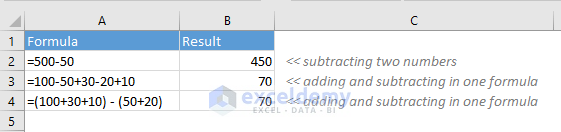## Adding and subtracting cell references in one formula

Suppose you want to subtract cell B2 from cell A2. In the cell C2, write a formula with these steps:

• At first, select cell C2
• Input an equal sign (=)
• Now select the cell reference A2
• Now input a minus sign (-)
• Then select the cell reference B2
• Now press Enter key on your keyboard. You will get the result.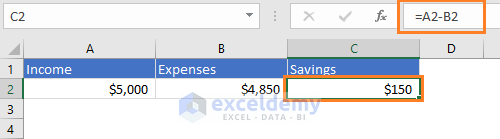## Subtract multiple cells from one cell in Excel

Method 1: Using the minus (-) sign

Suppose, in one cell (B1) you have entered your total Budget and in other cells (B2:B7), you have input your expenses (following image).

You can write a formula like the following one to find the Savings:

=B1-B2-B3-B4-B5-B6-B7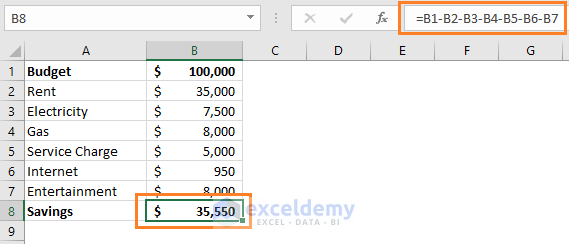But there is also an easier way. Check out my 2nd method.

Method 2: Using Excel’s SUM Function

In this method, at first, we have summed the cells of the range B2:B7 using Excel’s SUM function.

SUM(B2:B7)

Then we have subtracted the sum value from the cell B1.

B1- SUM(B2:B7)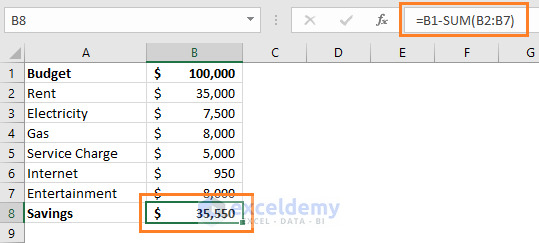## Using SUM function to add and subtract in one formula

In mathematics, subtracting a number from another number is same as summing a positive and a negative number.

For example, 50 – 20 and 50 + (-20) are actually the same thing.

In Excel, we can use this concept to add and subtract in one formula. Check out the following image.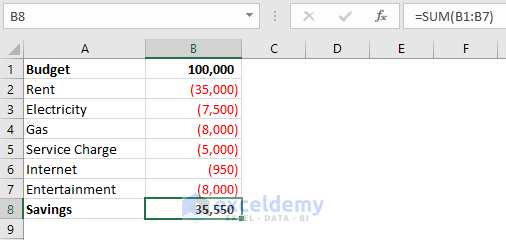In this way, we have used only the SUM function for the range B1:B7.

## Adding and subtracting two columns in one formula

Suppose you want to add the cells of the ranges B2:B7 and C2:C7 and then subtract the sum of the 2nd range from the first one. Here is the way (image below).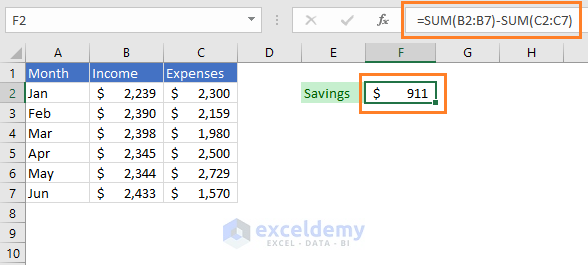## Subtracting percentage in Excel

It is easy to subtract two percentages values in Excel like:

100%-30%

=70%

Or you can also subtract percentages using cell references:

A2-B2; where A2 = 100% and B2 = 30%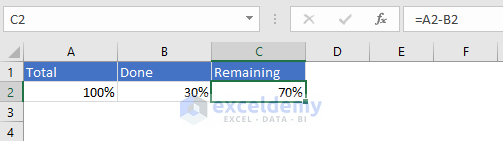## How to decrease a number by a certain percentage?

Suppose, your income is now \$5000 every month.

Due to a recession in your country, your employer has decreased salary by 30%.

You have to subtract the decreased percentage from 1. Then multiply the result with your original income. You will get your decreased income.

\$5000(1-30%)

= \$5000(1-0.3)

= \$5000 x 0.7

= \$3500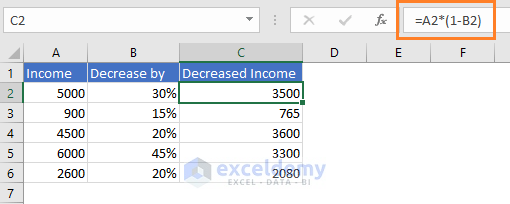## Conclusion

This is just a basic article on adding and subtracting. If you have any questions on this topic, let me know in the comment box.

Hello!
Welcome to my Excel blog! It took me some time to be a fan of Excel. But now I am a die-hard fan of MS Excel. I learn new ways of doing things with Excel and share here. Not only how to guide on Excel, but you will get also topics on Finance, Statistics, Data Analysis, and BI. Stay tuned!

1 Comment
1. ReplyQueda Apr 25, 2019 at 1:43 AM

I am trying to add and subtract across an Excel spreadsheet, ex. add cells B1, D1, F1 and then subtract the sum of cells C1, E1, G1. I thought I had it figured out, but . . . . this is what I came up with, but no go. I may be making this too hard! I can add columns, but can I not add across?
=SUM(B71,D71,F71,H71,J71,L71,N71,P71,R71)-SUM(C71,E71,G71,I71,K71,M71,O71,Q71,S71)